Get instant live expert help with Excel or Google Sheets“My Excelchat expert helped me in less than 20 minutes, saving me what would have been 5 hours of work!”

#### Post your problem and you’ll get expert help in seconds.

Your message must be at least 40 characters
Our professional experts are available now. Your privacy is guaranteed.

# Learn How to Get Data from Multiple Sheets into a Master SheetRead time: 15 minutes

You might have data in several Excel sheets that you need to be consolidated into one central location. Fortunately, you can to produce a Master Sheet with summaries and other totals from several different source sheets.

In this tutorial, we will introduce how to calculate totals and get summary data from multiple sheets with similar structure.

## Get data from multiple sheets into a master sheet in Excel

### 1. Calculate Total Based on Cell Reference

This article shows you how to get data from multiple sheets into a master sheet. Depending on the situation, this can be done by multiple methods. Let’s assume we have 3 sales sheets of 3 different stores: 1, 2 and 3 with similar formats below: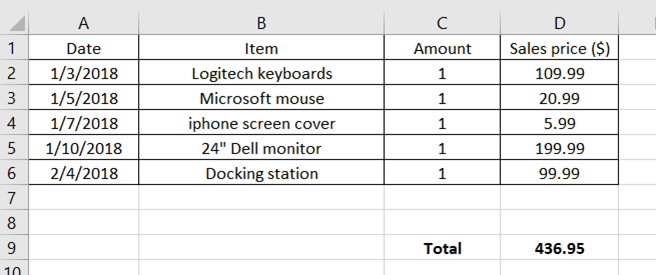We want to add the Total sales of Store 1, 2 and 3 together and put the result in a “Summary” sheet. In this example, we can use a cell reference as in the following formula: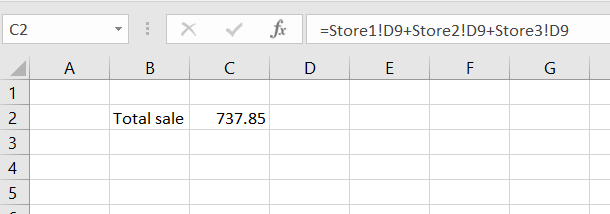The problem with using the addition operator is that we can’t keep adding cells with more sheets.

### 2. Calculate Totals Based on a 3D Formula

Excel has a 3D formula to help us sum all data across multiple adjacent sheets. On a Summary tab, type =sum( in cell C2, select cell D9 on Store1 tab, hold the Shift key and select the rest of the tab. The formula bar shows:

`=sum(‘Store1:Store3’!D9)`

This 3D formula calculates the total of all D9 cells in different sheets from Store 1 to Store 3.This formula gives the results the same as using cell reference. See below: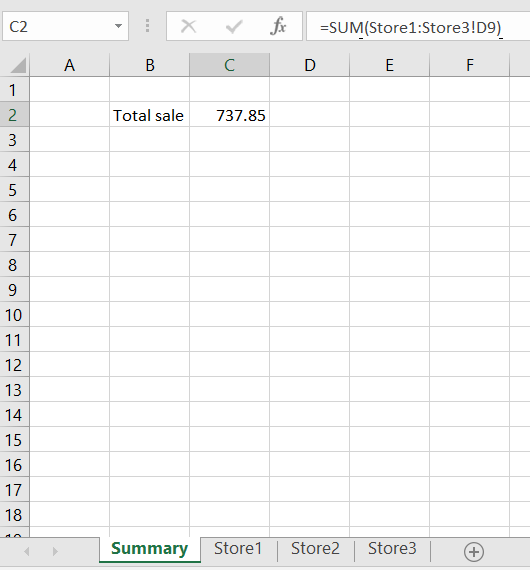Note when using this formula, the user must make sure to maintain the same order of the sheets. If any of the sheets in the middle of the sheet range (Store1 to Store3) is moved out of the range, that sheet will be excluded from the calculation. In the screenshot below, we see that when the Store2 sheet is moved to the right of the Store3 sheet, the Total sale value drops to 645.9 which is Store1 + Store2 sales only.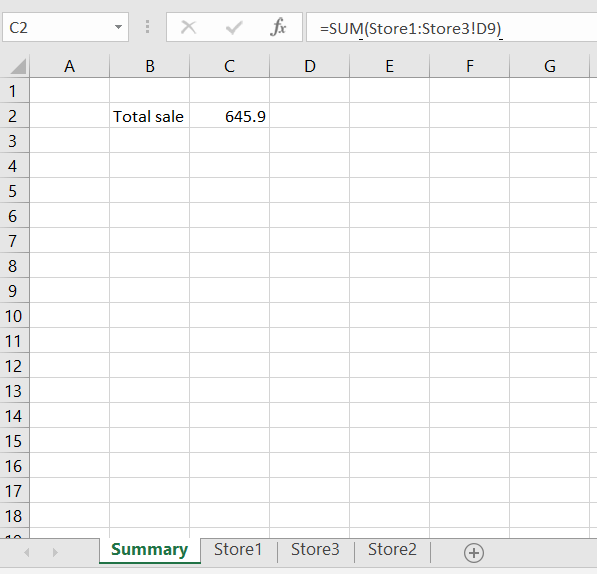### 3.Gather data from multiple sheets using INDIRECT()

We can add data from multiple sheets into a summary sheet using INDIRECT() function. INDIRECT() function need us to store the name of the sheet explicitly.

`=SUM(INDIRECT(C1&"!"&"D9"),INDIRECT(D1&"!"&"D9"),INDIRECT(E1&"!"&"D9"))`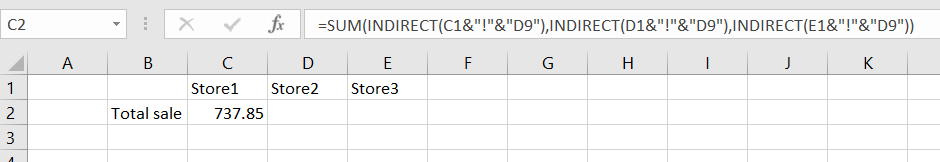In the formula above, INDIRECT(C1&”!”&”D9″) gives us the total sales located at cell D9 on Store1 sheet, INDIRECT(D1&”!”&”D9″) gives us the total sales located at cell D9 on Store2, and so on.

This formula is better than the 3D formula because it does not depend on the sheet location. However, the formula above requires a list of all the sheet names.

As you have seen from this tutorial, gathering data from multiple sheets can be done in several different ways and there is no best solution. Depending on the situation, one can use 3D formula or INDIRECT() function. In some other cases, you can also use Data Consolidation to calculate the total.

Different problems have their own best solution. If you are stuck and want to get to the solution quick, ask your question to have it answered by an Excel expert in 20 minutes. They are available to help you 24/7 at the link to the right. The first question is free.

### Did this post not answer your question? Get a solution from connecting with the expert.Another blog reader asked this question today on Excelchat:
Related blogs
Solution examplesI have a google sheet that takes information from a google form, that creates a row every time a form is submitted. Since it is a new row that is created, rather than filling in the next row down, the formulas I have applied to some of the columns do not work. The same is true when I try and link the data to another sheet to bypass this problem. This is probably quite a general functionality issue so I won't send the actual document but let me know if you need it
Solved by F. F. in 13 minsHello, I need help regarding indirect formula of excel. 1. What is the purpose of using this formula? 2. If we use this formula with sum formula, then why we are using this with sum formula, we can also perform addition from different sheets without indirect formula ?
Solved by O. D. in 21 minsI want to dynamic access a sheet/cell from a closed file using INDEX. But when I try to "&A3&" for sheet name I forces me to select a file and then populates the sheet name instead of making it dynamically INDEX('/Users/me/Desktop/["&A2&"]"&A3&"'!A:A,1,1)
Solved by G. H. in 20 minsI need explanation for the excel formula below... =IF(ISERROR(SUMIFS(INDIRECT("'"&BE\$6&"'!"&"\$G:\$G"),INDIRECT("'"&BE\$6&"'!"&"\$R:\$R"),\$A39,INDIRECT("'"&BE\$6&"'!"&"\$F:\$F"),"<>52711",INDIRECT("'"&BE\$6&"'!"&"\$C:\$C"),10)),0,SUMIFS(INDIRECT("'"&BE\$6&"'!"&"\$G:\$G"),INDIRECT("'"&BE\$6&"'!"&"\$R:\$R"),\$A39,INDIRECT("'"&BE\$6&"'!"&"\$F:\$F"),"<>52711",INDIRECT("'"&BE\$6&"'!"&"\$C:\$C"),10))+IF(ISERROR(SUMIFS(INDIRECT("'"&BE\$4&"'!"&"\$A\$30:\$EO\$30"),INDIRECT("'"&BE\$4&"'!"&"\$A\$4:\$EO\$4"),BE\$5,INDIRECT("'"&BE\$4&"'!"&"\$A\$5:\$EO\$5"),\$A39)),0,SUMIFS(INDIRECT("'"&BE\$4&"'!"&"\$A\$30:\$EO\$30"),INDIRECT("'"&BE\$4&"'!"&"\$A\$4:\$EO\$4"),BE\$5,INDIRECT("'"&BE\$4&"'!"&"\$A\$5:\$EO\$5"),\$A39))+IF(ISERROR(SUMIF(INDIRECT("'"&BE\$6&" "&\$A\$31&"'!"&"H:H"),\$A39,(INDIRECT("'"&BE\$6&" "&\$A\$31&"'!"&"I:I")))),0,SUMIF(INDIRECT("'"&BE\$6&" "&\$A\$31&"'!"&"H:H"),\$A39,(INDIRECT("'"&BE\$6&" "&\$A\$31&"'!"&"I:I"))))
Solved by V. A. in 20 mins## Subscribe to Excelchat.coAnother blog reader asked this question today on Excelchat: